# Sinc-squared function

This article is about a particular function from a subset of the real numbers to the real numbers. Information about the function, including its domain, range, and key data relating to graphing, differentiation, and integration, is presented in the article.
View a complete list of particular functions on this wiki
For functions involving angles (trigonometric functions, inverse trigonometric functions, etc.) we follow the convention that all angles are measured in radians. Thus, for instance, the angle of$90\,^\circ$ is measured as$\pi/2$.

## Definition

This function is defined as the composite of the square function and the sinc function. Explicitly, it is given as: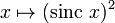$x \mapsto (\operatorname{sinc} \ x)^2$

Alternatively, it is given as:$\operatorname{sinc}^2 x := \left\lbrace \begin{array}{rl} 1, & x = 0 \\ \frac{\sin^2x}{x^2}, & x \ne 0 \\\end{array}\right.$

## Key data

Item Value
default domain all real numbers, i.e., all of$\R$
range the closed interval$[0,1]$, i.e., the set$\{ y \mid 0 \le y \le 1 \}$
absolute maximum value: 1, absolute minimum value: 0
period none; the function is not periodic
horizontal asymptotes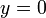$y = 0$, i.e., the$x$-axis. This is because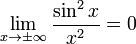$\lim_{x \to \pm \infty} \frac{\sin^2 x}{x^2} = 0$, which in turn can be deduced from the fact that the numerator is bounded while the magnitude of the denominator approaches$\infty$.
local maximum values and points of attainment The local maximum values occur at all points where$\tan^2x = x^2$. There is an anomalous local maximum at$x = 0$ with value 1. All other local maxima occur at points close to odd multiples of$\pi/2$ (except$\pm \pi/2$ themselves, though not exactly at those points.
local minimum values and points of attainment The local minimum values occur at all points where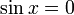$\sin x = 0$ except the point$x = 0$ itself. These are precisely the nonzero integer multiples of$\pi$. The values are all equal to zero.
derivative Fill this in later
second derivative Fill this in later
antiderivative$\operatorname{Si}(2x) - \frac{\sin^2x}{x} + C$. See #First antiderivative. Here$\operatorname{Si}$ denotes the sine integral, an antiderivative for the sinc function.
power series and Taylor series The power series about 0 (which is also the Taylor series) is Fill this in later

## Graph

Here is a graph on the interval$[-3\pi,3\pi]$:

The graph is a little unclear, here is an alternative version where different scalings are used for the$x$-axis and$y$-axis:

## Integration

### First antiderivative

Denote by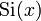$\operatorname{Si}(x)$ the function$\int_0^x \operatorname{sinc} t \, dt = \int_0^x (\sin t)/t \, dt$. We integrate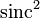$\operatorname{sinc}^2$ and obtain the following answer in terms of$\operatorname{Si}$: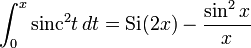$\int_0^x \operatorname{sinc}^2t \, dt = \operatorname{Si}(2x) - \frac{\sin^2x}{x}$

For the indefinite integral, we can put a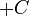$+C$ at the end..

We do this using integration by parts: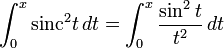$\int_0^x \operatorname{sinc}^2t \, dt = \int_0^x \frac{\sin^2t}{t^2} \, dt$

Take$1/t^2 \, dt$ as the part to integrate. We get: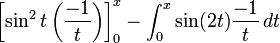$\left[\sin^2t\left(\frac{-1}{t}\right)\right]_0^x - \int_0^x \sin(2t) \frac{-1}{t} \, dt$

This becomes:$\frac{-\sin^2x}{x} + \lim_{t \to 0} \frac{\sin^2t}{t} + \int_0^x \frac{\sin(2t)}{t} \, dt$

The limit expression is zero because$\sin^2$ has a zero of order 2 at zero. For the integration expression, set$u = 2t$ and get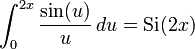$\int_0^{2x} \frac{\sin(u)}{u} \, du = \operatorname{Si}(2x)$. Plugging back in, we get the desired answer.

### Improper definite integral

We know that:$\int_0^\infty \operatorname{sinc} t \, dt = \lim_{x \to \infty} \operatorname{Si}(x) = \frac{\pi}{2}$

Using this, we obtain that: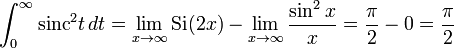$\int_0^\infty \operatorname{sinc}^2 t \,dt = \lim_{x \to \infty} \operatorname{Si}(2x) - \lim_{x \to \infty} \frac{\sin^2x}{x} = \frac{\pi}{2} - 0 = \frac{\pi}{2}$

Similarly: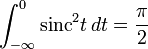$\int_{-\infty}^0 \operatorname{sinc}^2 t \, dt = \frac{\pi}{2}$

Overall:$\int_{-\infty}^\infty \operatorname{sinc}^2 t \, dt = \pi$

Note that the improper integral value is the same for the sinc function and its square. Roughly speaking, the sinc function is bigger than its square when both are positive, but the sinc function also takes negative values while its square does not, and so these differences balance out in the overall integration.